# AP Physics B : Using Torque Equations

## Example Questions

### Example Question #1 : Using Torque Equations

A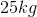child sits on one end of aseesaw. How far from the pivot point should a rock of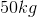be placed on the other side of the seesaw in order to perfectly balance the child and rock?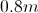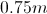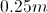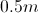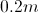Explanation:

This problem requires us to add torques about the pivot point. In order for the seesaw to be balanced, the torque must be equal on each side of the pivot point.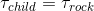Use the equation for torque in this equation.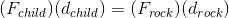The force of each object will be equal to the force of gravity.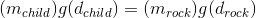Gravity can be canceled from each side of the equation. for simplicity.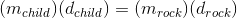Now we can use the mass of the rock and the mass of the child. The total length of the seesaw is two meters, and the child sits at one end. The child's distance from the center of the seesaw will be one meter.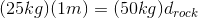Solve for the distance between the rock and the center of the seesaw.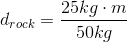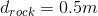### Example Question #2 : Using Torque Equations

A uniform metal bar with massand length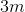is connected to a wall by a pivot joint. It is held in place by an ideal thin cable (no effective mass or elasticity) connected to the wall above the pivot, so that the cable makes aangle with the metal bar. What is the tension in the cable?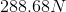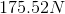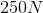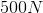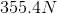Explanation:

The metal bar is in rotational equilibrium, meaning there is no net torque. Use the wall pivot as the rotation point to eliminate having to calculate torque due to the unknown wall force, since the wall exerts no torque around the pivot. This leaves the rope tension and the weight of the bar as the only torque generators.

Calculate the torques of those forces around the wall pivot with a standard procedure:

1)  Draw in the force vector arrows on the beam at their points of application.

2)  Draw a line through the force arrows extending to the edges of the drawing. This is the “line of force.”

3)  Draw a line perpendicular to the line of force from the axis of rotation (the wall pivot, in this case) to the line of force at the point where it is nearest the axis of rotation. This is the “perpendicular lever arm.”

The torque is then the force applied times its perpendicular lever arm around the axis of rotation.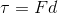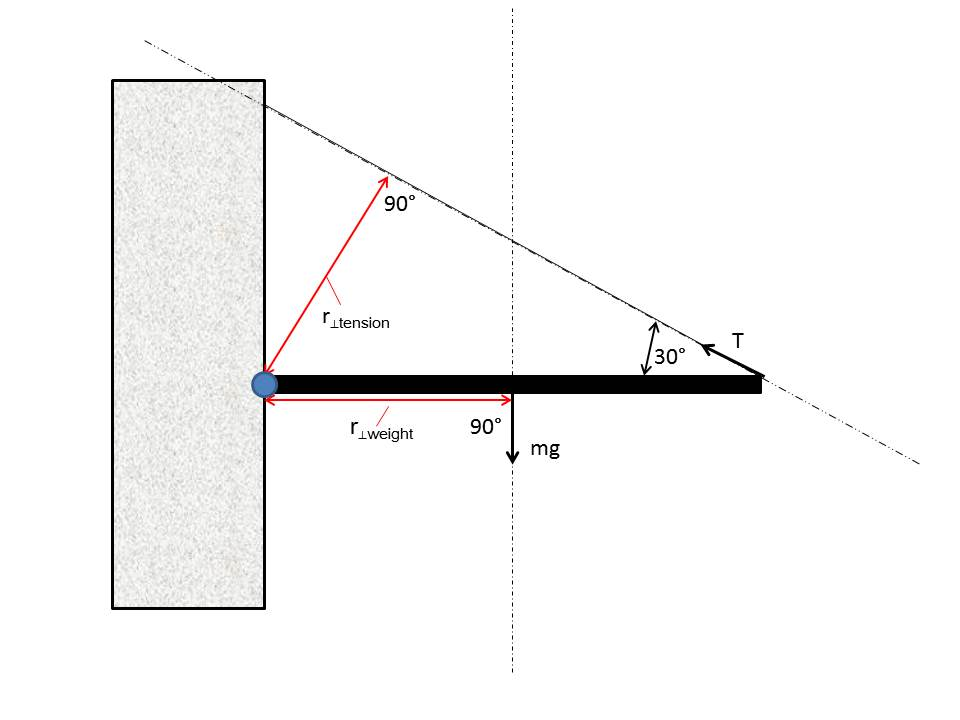Consider, for a uniform bar, the weight being applied at the center. The closest approach of the weight’s line of force to the pivot point is the center of the bar; hence, half the bar’s length is the perpendicular lever arm. So the torque of the weight,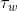, around the pivot point is: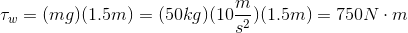The tension is applied at the end of the bar, at aangle with respect to the bar. The perpendicular lever arm can be calculated trigonometrically: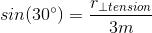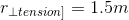We can then find the torque generated by the tension force of the cable:These torques produce rotations in the opposite direction, which is why they can cancel out to cause static equilibrium. Using the convention that counterclockwise rotations (as viewed in the diagram, with the wall on the left) are positive, the case of static equilibrium gives: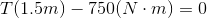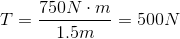For this configuration, the tension in the cable happens to equal the weight of the bar.